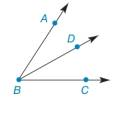Chapter 1.4, Problem 5E### Elementary Geometry for College St...

6th Edition
Daniel C. Alexander + 1 other
ISBN: 9781285195698

#### Solutions

Chapter
Section### Elementary Geometry for College St...

6th Edition
Daniel C. Alexander + 1 other
ISBN: 9781285195698
Textbook Problem
1 views

# In Exercises 5 to 8, describe in one word the relationship between the angles. ∠ A B D and ∠ D B CTo determine

To describe:

The relationship between the given angles.

Explanation

Given:

The given angles are, ABD and DBC.

The given figure is,

### Still sussing out bartleby?

Check out a sample textbook solution.

See a sample solution

#### The Solution to Your Study Problems

Bartleby provides explanations to thousands of textbook problems written by our experts, many with advanced degrees!

Get Started

#### Evaluate the definite integral. 0axx2+a2dx(a0)

Single Variable Calculus: Early Transcendentals, Volume I

#### let f(x) = x 1, g(x) = x+1, and h(x) = 2x3 1. Find the rule for each function. 17. f-hg

Applied Calculus for the Managerial, Life, and Social Sciences: A Brief Approach

#### Minimize Subject to C=4x+2y+6z x+2y+z42x+y+2z23x+2y+z3x0,y0,z0

Finite Mathematics for the Managerial, Life, and Social Sciences

#### = _____. 0 does not exist

Study Guide for Stewart's Single Variable Calculus: Early Transcendentals, 8th